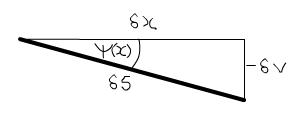#### You may also like### Overarch 2

Bricks are 20cm long and 10cm high. How high could an arch be built without mortar on a flat horizontal surface, to overhang by 1 metre? How big an overhang is it possible to make like this?### Stonehenge

Explain why, when moving heavy objects on rollers, the object moves twice as fast as the rollers. Try a similar experiment yourself.### Maximum Flow

Given the graph of a supply network and the maximum capacity for flow in each section find the maximum flow across the network.

# Beam Me Up

##### Age 16 to 18 Challenge Level:

Like Mach Attack, this question provides an opportunity to derive an interesting engineering formula for yourself. You might see it quite quickly, but don't be too concerned if you don't.

Understanding the formula $\psi = \int \kappa ds$

Carrying on from the hint, $\int_{s=0}^{\phi r} \kappa ds = \int_{0}^{\phi r} \frac{1}{r} ds = \phi$, the angle swept out at the centre of the circle. If we then draw a straight line from $s=0$ to $s=\phi r$, we find that $\phi = \psi$, where $\psi$ is the angle the beam has deviated from a straight line. Thus the formula is proved for circles. For a more general curve, as we zoom in around a point, we can approximate the curve better and better by a circle, until in the limit of zooming in to a single point it matches exactly.

Find $v$ in termsof $\kappa$ and $x$

Deriving the formulae in this problem is all to do with looking at small limits, i.e. considering a distance $\delta s$ along the curve which is very small. This is a common trick in engineering, and at the end we will integrate over all of these small sections to get the full result.

When $\delta s$ is small, we can approximate the situation by a triangle:[Note about the minus sign in the above diagram: this is simply convention that vertical displacement is positive up, but curvature and angle are positive when the beam is bent down, such that the top of the beam is in tension.]

Thus $- \delta v = \delta s \sin \psi (x)$.

For small deflections, such as we are considering, $\psi$ is small so $\sin\psi \approx \psi$ and $\delta x = \delta s \cos \psi \approx \delta s$. Thus $- \delta v \approx \delta x \psi (x)$.

To get total deflection we need to sum all these small deflections along the length of the beam, which is where integrating comes in. We find $v = - \int{\psi}dx$

And since $\psi = \int{\kappa}dx$, we find the formula $v = - \int{\int{\kappa}}dx dx$. You could alternatively write this as $\kappa = -\frac{d^2v}{dx^2}$.

You can apply this formula to a real problem in Euler's Buckling Formula.